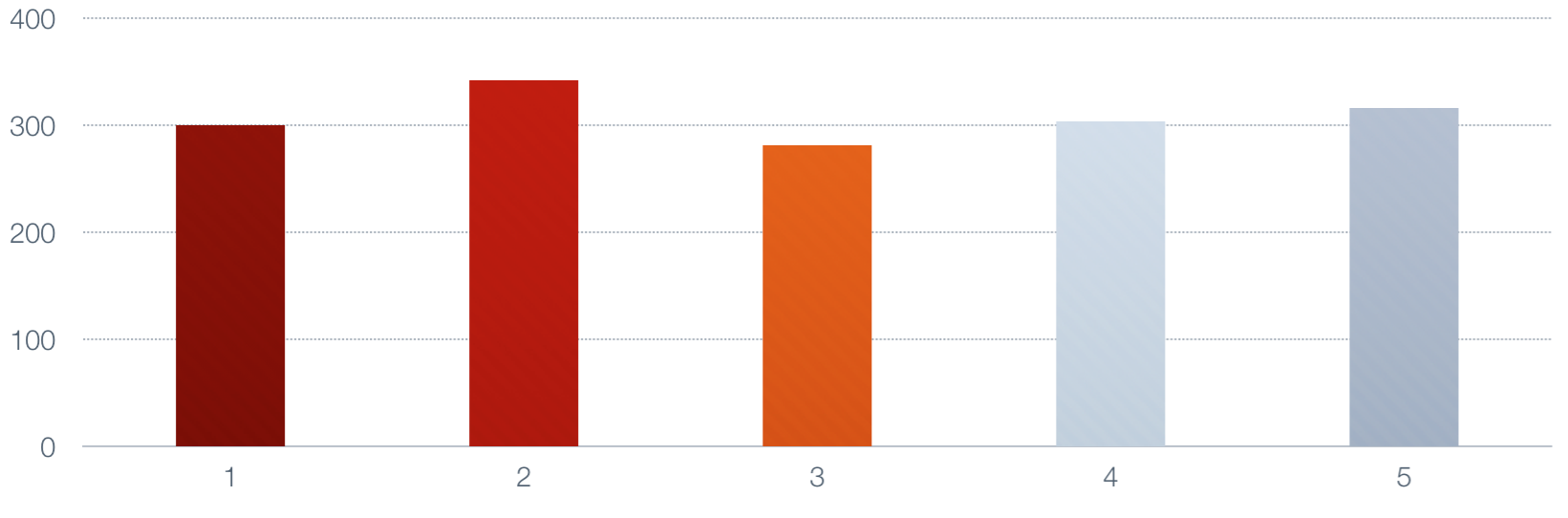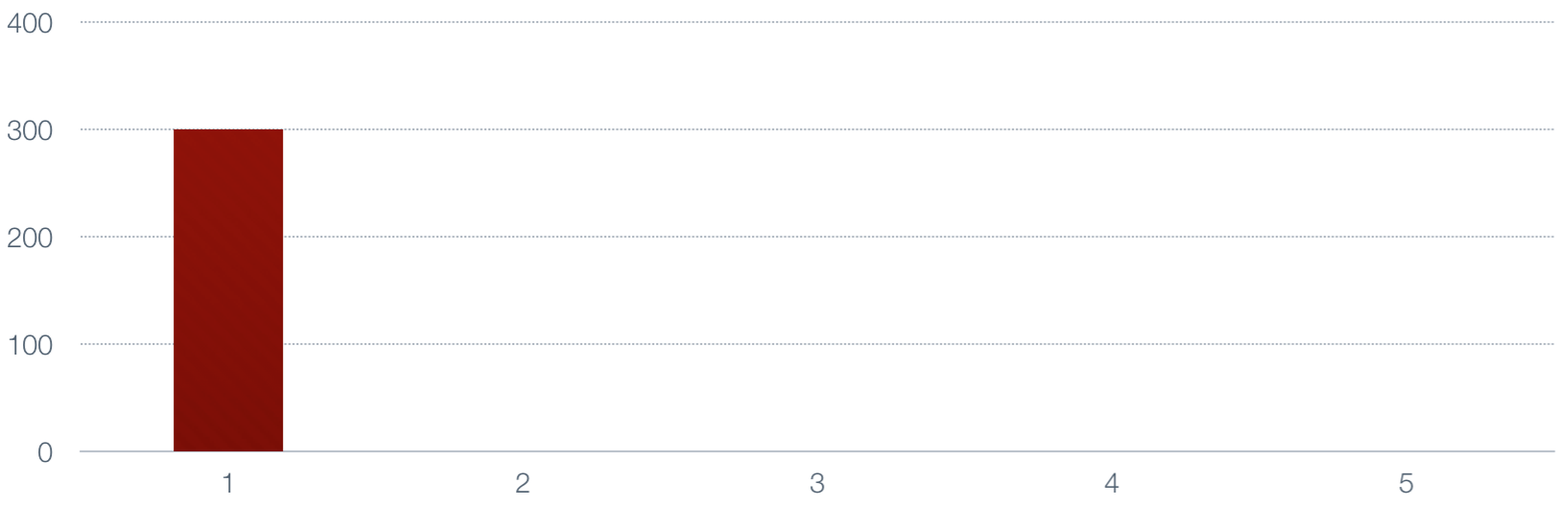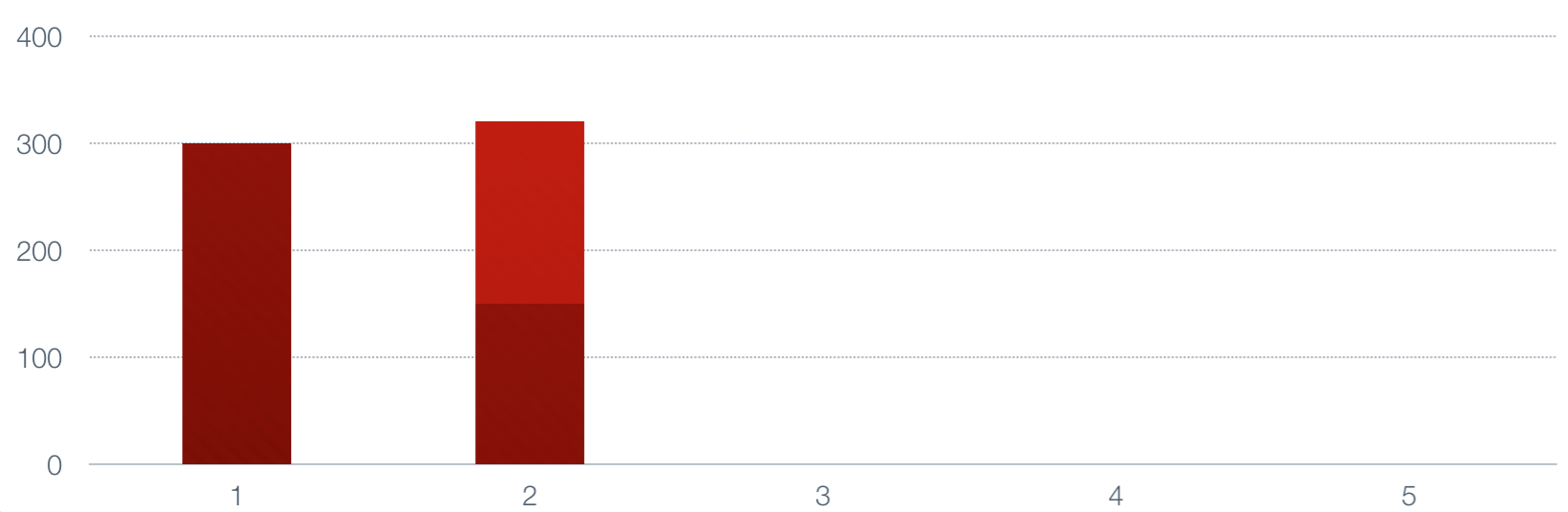Popularity
6.4
Growing
Activity
0.0
Declining
407
25
33

Programming language: Go

# ewma alternatives and similar packages

Based on the "Science and Data Analysis" category.
Alternatively, view ewma alternatives based on common mentions on social networks and blogs.

• ### gonum

Gonum is a set of numeric libraries for the Go programming language. It contains libraries for matrices, statistics, optimization, and more
• ### Stats

A well tested and comprehensive Golang statistics library package with no dependencies.
• ### Static code analysis for 29 languages.

Your projects are multi-language. So is SonarQube analysis. Find Bugs, Vulnerabilities, Security Hotspots, and Code Smells so you can release quality code every time. Get started analyzing your projects today for free.
• ### gonum/plot

A repository for plotting and visualizing data
• ### gosl

Linear algebra, eigenvalues, FFT, Bessel, elliptic, orthogonal polys, geometry, NURBS, numerical quadrature, 3D transfinite interpolation, random numbers, Mersenne twister, probability distributions, optimisation, differential equations.
• ### streamtools

tools for working with streams of data
• ### dataframe-go

DataFrames for Go: For statistics, machine-learning, and data manipulation/exploration
• ### go-dsp

Digital Signal Processing for Go
• ### chart

Provide basic charts in go
• ### goraph

Package goraph implements graph data structure and algorithms.
• ### graph

Graph algorithms and data structures
• ### gonum/mat64

The general purpose package for matrix computation. Package mat64 provides basic linear algebra operations for float64 matrices.
• ### go.matrix

linear algebra for go
• ### calendarheatmap

📅 Calendar heatmap inspired by GitHub contribution activity
• ### gohistogram

Streaming approximate histograms in Go
• ### TextRank

:wink: :cyclone: :strawberry: TextRank implementation in Golang with extendable features (summarization, phrase extraction) and multithreading (goroutine).
• ### sparse

Sparse matrix formats for linear algebra supporting scientific and machine learning applications
• ### blas

Go implementation of BLAS (Basic Linear Algebra Subprograms)
• ### pagerank

Weighted PageRank implementation in Go
• ### go-estimate

State estimation and filtering algorithms in Go
• ### geom

2d geometry for golang
• ### vectormath

Vectormath for Go
• ### evaler

Implements a simple floating point arithmetic expression evaluator in Go (golang).
• ### jsonl-graph

🏝 JSONL Graph Tools
• ### gostat

Collection of statistical routines in golang
• ### triangolatte

2D triangulation library. Allows translating lines and polygons (both based on points) to the language of GPUs.
• ### permutation

Simple permutation package for golang
• ### goent

GO Implementation of Entropy Measures
• ### piecewiselinear

tiny linear interpolation library for go (factored out from https://github.com/sgreben/yeetgif)
• ### PiHex

PiHex Library, written in Go, generates a hexadecimal number sequence in the number Pi in the range from 0 to 10,000,000.
• ### ode

An ordinary differential equation solving library in golang.
• ### gograph

A golang generic graph library that provides mathematical graph-theory and algorithms.
• ### GoStats

GoStats is a go library for math statistics mostly used in ML domains, it covers most of the statistical measures functions.
• ### rootfinding

root-finding library
• ### godesim

ODE system solver made simple. For IVPs (initial value problems).
• ### assocentity

Package assocentity returns the mean distance from tokens to an entity and its synonyms
• ### gofrac

A fractions library for go (http://golang.org)

Package to do Bradley-Terry Model pairwise compairsons

• ### gobbs

A Blum-Blum-Shub-Generator written in Go

• ### mudlark-go

A collection of packages providing (hopefully) useful code for use in software using Google's Go programming language.

Do you think we are missing an alternative of ewma or a related project?

## EWMAThis repo provides Exponentially Weighted Moving Average algorithms, or EWMAs for short, based on our Quantifying Abnormal Behavior talk.

### Exponentially Weighted Moving Average

An exponentially weighted moving average is a way to continuously compute a type of average for a series of numbers, as the numbers arrive. After a value in the series is added to the average, its weight in the average decreases exponentially over time. This biases the average towards more recent data. EWMAs are useful for several reasons, chiefly their inexpensive computational and memory cost, as well as the fact that they represent the recent central tendency of the series of values.

The EWMA algorithm requires a decay factor, alpha. The larger the alpha, the more the average is biased towards recent history. The alpha must be between 0 and 1, and is typically a fairly small number, such as 0.04. We will discuss the choice of alpha later.

The algorithm works thus, in pseudocode:

1. Multiply the next number in the series by alpha.
2. Multiply the current value of the average by 1 minus alpha.
3. Add the result of steps 1 and 2, and store it as the new current value of the average.
4. Repeat for each number in the series.

There are special-case behaviors for how to initialize the current value, and these vary between implementations. One approach is to start with the first value in the series; another is to average the first 10 or so values in the series using an arithmetic average, and then begin the incremental updating of the average. Each method has pros and cons.

It may help to look at it pictorially. Suppose the series has five numbers, and we choose alpha to be 0.50 for simplicity. Here's the series, with numbers in the neighborhood of 300.Now let's take the moving average of those numbers. First we set the average to the value of the first number.Next we multiply the next number by alpha, multiply the current value by 1-alpha, and add them to generate a new value.This continues until we are done.Notice how each of the values in the series decays by half each time a new value is added, and the top of the bars in the lower portion of the image represents the size of the moving average. It is a smoothed, or low-pass, average of the original series.

For further reading, see Exponentially weighted moving average on wikipedia.

### Choosing Alpha

Consider a fixed-size sliding-window moving average (not an exponentially weighted moving average) that averages over the previous N samples. What is the average age of each sample? It is N/2.

Now suppose that you wish to construct a EWMA whose samples have the same average age. The formula to compute the alpha required for this is: alpha = 2/(N+1). Proof is in the book "Production and Operations Analysis" by Steven Nahmias.

So, for example, if you have a time-series with samples once per second, and you want to get the moving average over the previous minute, you should use an alpha of .032786885. This, by the way, is the constant alpha used for this repository's SimpleEWMA.

### Implementations

This repository contains two implementations of the EWMA algorithm, with different properties.

The implementations all conform to the MovingAverage interface, and the constructor returns that type.

Current implementations assume an implicit time interval of 1.0 between every sample added. That is, the passage of time is treated as though it's the same as the arrival of samples. If you need time-based decay when samples are not arriving precisely at set intervals, then this package will not support your needs at present.

#### SimpleEWMA

A SimpleEWMA is designed for low CPU and memory consumption. It will have different behavior than the VariableEWMA for multiple reasons. It has no warm-up period and it uses a constant decay. These properties let it use less memory. It will also behave differently when it's equal to zero, which is assumed to mean uninitialized, so if a value is likely to actually become zero over time, then any non-zero value will cause a sharp jump instead of a small change.

#### VariableEWMA

Unlike SimpleEWMA, this supports a custom age which must be stored, and thus uses more memory. It also has a "warmup" time when you start adding values to it. It will report a value of 0.0 until you have added the required number of samples to it. It uses some memory to store the number of samples added to it. As a result it uses a little over twice the memory of SimpleEWMA.

## Usage

### API Documentation

View the GoDoc generated documentation here.

``````package main

import "github.com/VividCortex/ewma"

func main() {
samples := float64{
4599, 5711, 4746, 4621, 5037, 4218, 4925, 4281, 5207, 5203, 5594, 5149,
}

e := ewma.NewMovingAverage()  //=> Returns a SimpleEWMA if called without params
a := ewma.NewMovingAverage(5) //=> returns a VariableEWMA with a decay of 2 / (5 + 1)

for _, f := range samples {
}

e.Value() //=> 13.577404704631077
a.Value() //=> 1.5806140565521463e-12
}
``````

## Contributing

We only accept pull requests for minor fixes or improvements. This includes:

• Small bug fixes
• Typos